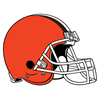FanDuel 2019 AFC Championship Odds
-110
0.91 to 1Ravens
45.4% implied probability

+260
2.6 to 1Patriots
24.1% implied probability

+480
4.8 to 1Chiefs
14.9% implied probability

+2100
21 to 1Bills
3.9% implied probability

+2300
23 to 1Texans
3.6% implied probability

+2300
23 to 1Titans
3.6% implied probability

+3900
39 to 1Steelers
2.2% implied probability

+25000
250 to 1Jets
0.3% implied probability

+25000
250 to 1Raiders
0.3% implied probability

+25000
250 to 1Browns
0.3% implied probability

+25000
250 to 1Colts
0.3% implied probability

+25000
250 to 1Chargers
0.3% implied probability

+35000
350 to 1Broncos
0.2% implied probability

+40000
400 to 1Jaguars
0.2% implied probability

+200000
2000 to 1Bengals
0.0% implied probability

+300000
3000 to 1Dolphins
0.0% implied probability